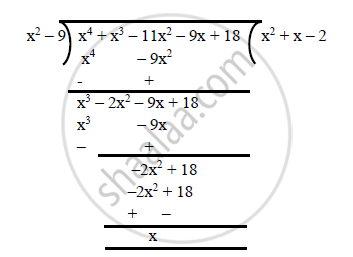# If 3 and –3 Are Two Zeroes of the Polynomial (X^4 + X^3 – 11x^2 – 9x + 18), Find All the Zeroes of the Given Polynomial. - Mathematics

If 3 and –3 are two zeroes of the polynomial (x^4 + x^3 – 11x^2 – 9x + 18), find all the zeroes of the given polynomial.

#### Solution

Let x^4 + x^3 – 11x^2 – 9x + 18
Since 3 and – 3 are the zeroes of f(x), it follows that each one of (x + 3) and (x – 3) is a factor of f(x).
Consequently, (x – 3) (x + 3) = (x^2 – 9) is a factor of f(x).
On dividing f(x)   by  (x^2 – 9), we get:f(x) = 0 ⇒ (x^2 + x – 2) (x^2 – 9) = 0
⇒ (x^2 + 2x – x – 2) (x – 3) (x + 3)
⇒ (x – 1) (x + 2) (x – 3) (x + 3) = 0
⇒ x = 1 or x = -2 or x = 3 or x = -3
Hence, all the zeroes are 1, -2, 3 and -3.

Concept: Relationship Between Zeroes and Coefficients of a Polynomial
Is there an error in this question or solution?

#### APPEARS IN

RS Aggarwal Secondary School Class 10 Maths
Chapter 2 Polynomials
Exercises 2 | Q 14
Share